### Nano Res.[理论]│崔小强等:应用机器学习快速筛选过渡金属单原子负载N掺杂γ相石墨炔用于一氧化碳电还原C1产物的理论研究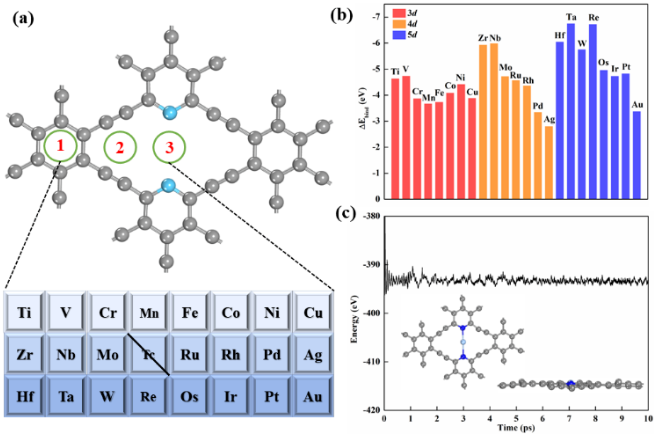Figure1 (a) The considered three sites for TM anchored on N-GY (b) Binding energies of TM anchored on N-GY. (c) Energy profile of AIMD simulation on Ag@N-GY at 500 K.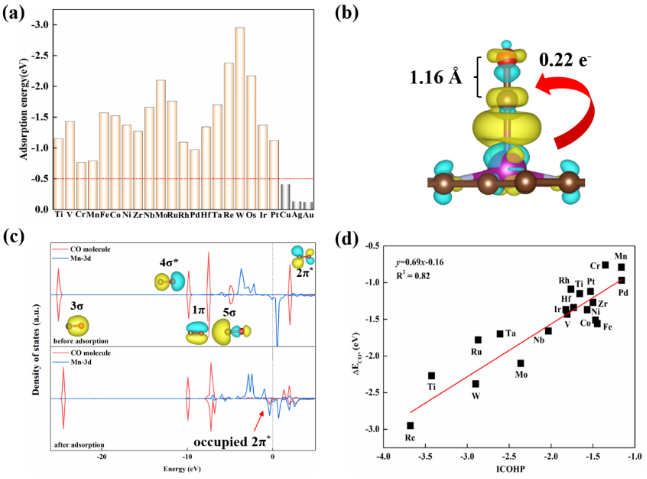Figure2 (a)The computed adsorption energy of CO on TM@N-GY.(b) The computed charge density differenceof CO adsorption on Mn@N-GY. (c) The partial density of states (PDOSs) between CO and Mn before and after CO adsorption. (d) The correlation between integrated crystal orbital Hamilton population (ICOHP) and the adsorption energy (ΔEads) of CO.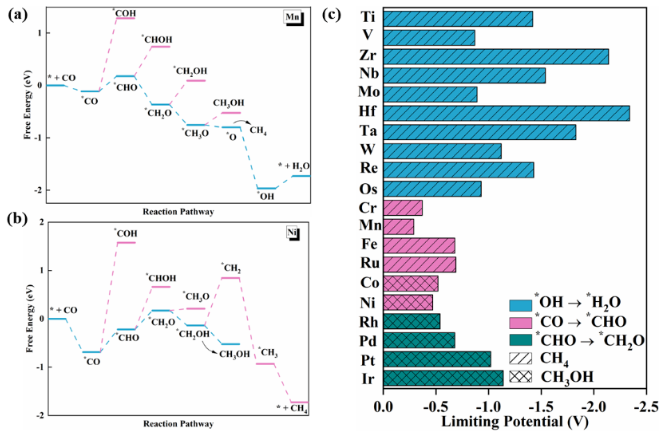Figure3 (a) Gibbs free energy profiles for CO reduction to CH4 on Mn@N-GY. (b) Gibbs free energy profiles for CO reduction to CH3OH on Ni@N-GY.(c)Computed theoretical limiting potential (UL) on twenty catalysts ofTM@N-GY.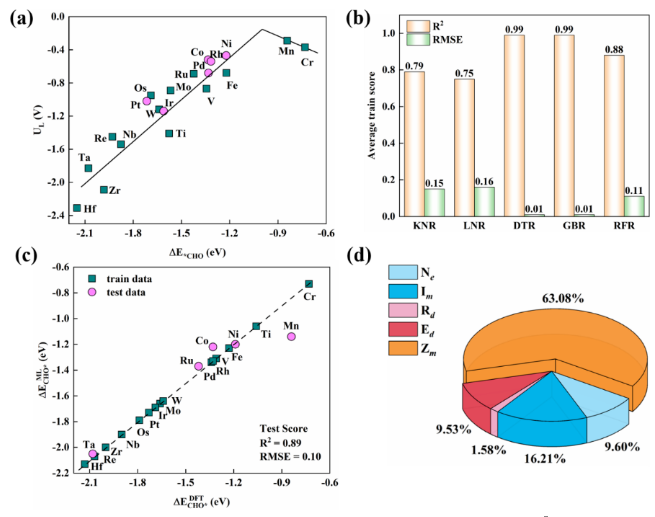Figure 4 (a) Volcano plots between ∆ECHO* and UL. (b) The RMSE and the Rscore on the test set of machine learning models with different algorithms for ∆ECHO*. (c) Comparison between DFT-calculated and ML-predicted ∆ECHO*. Green and pink points correspond to the training data and test data, respectively. (d) Pie chart for feature importance analysis in the ML model.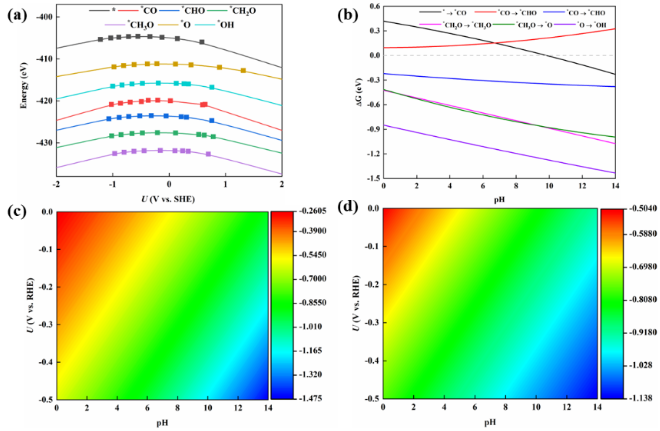Figure5 (a) Computed energies of the Mn@N-GY andcorresponding reaction intermediates as a function of the applied electrode potential (SHE). (b) Free energy changes of the reaction steps of Mn@N-GY as a function of pH. (c) pH-dependent and potential-dependent contour plot of adsorption energies of*CO and (d) *CHO on Mn@N-GY.

Jiao D, Zhang D, Wang D, et al. Applying machine-learning screening of single transition metal atoms anchored on N-doped γ-graphyne for carbon monoxide electroreduction toward C1 products. Nano Research, 2023, https://doi.org/10.1007/s12274-023-5773-0.down
wechat